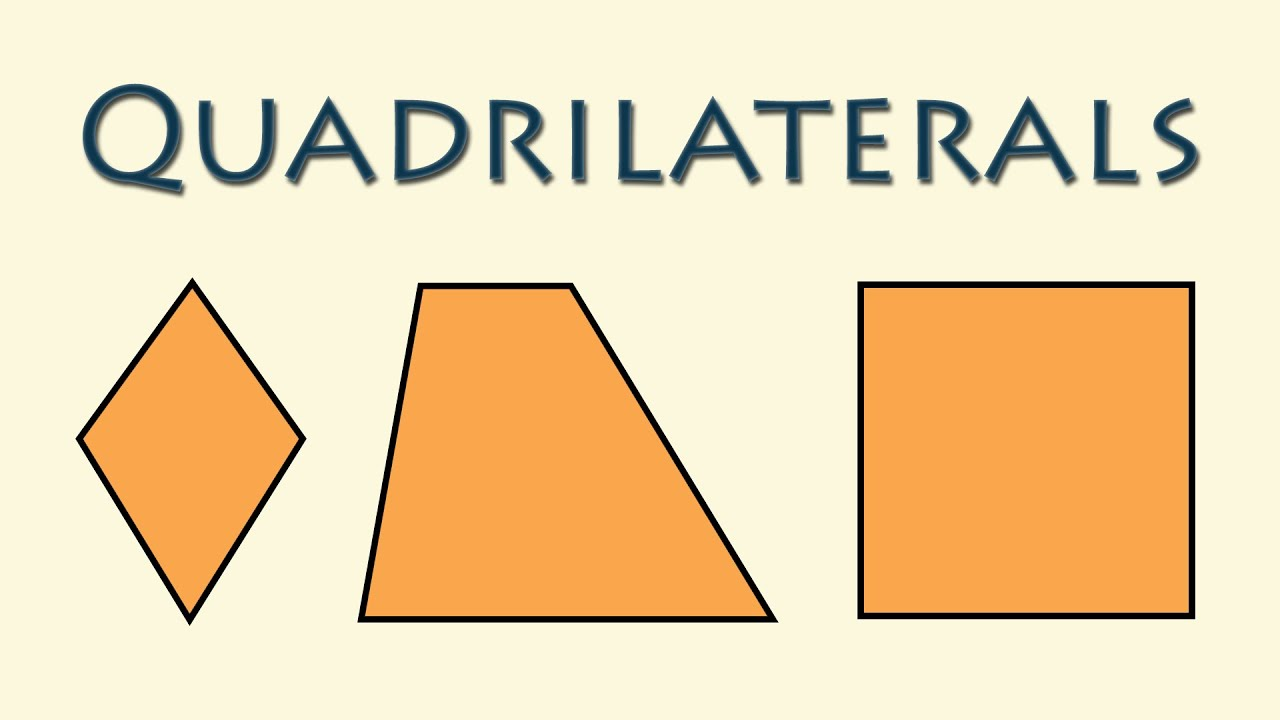## Thursday, May 23, 2019What happens when you make a quadrilateral (a four sided object) and connect the midpoints of each side?  What if you did this over and over again?

Here is an activity that investigates that!

1. Draw any quadrilateral that takes up most of the page.  Measure the interior angles and add them all together and record this.  Measure and record the perimeter
2. Measure and determine the midpoint of each line.  Connect the midpoints and make a new quadrilateral.  (Iteration 1) Measure the interior angles of the new quadrilateral and perimeter.
3. Repeat step 2, and fill in the chart with the sum of the new angles and perimeter.
4. Estimate what the sum of the angles and perimeter will be the for 10th shape.  (Do not make the 10th shape, simply estimate based on the pattern).
5. Color in your shape to make a creative design.

What do you notice?  Wonder? What would be the sum of the interior angles of iteration 10?  Perimeter?

Here is a recording sheet.

A possible example:

Extension:

Simply show this animation and ask "What is this visually proving?"A possible video to show is here: